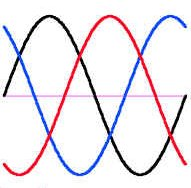# Packet Power BlogLet’s use our water pipe analogy again: imagine an “AC water” system that delivers alternating pulses of water pressure and vacuum in a closed loop system, using two pipes. Water flows to the receiver (some sort of a hydraulic engine) through one pipe (lets call it A), then back to the source through another pipe (lets call it B). Every few seconds or so the direction of water flow reverses.

Now imagine that you wanted to deliver three times as much power. You would need three such systems (six pipes total, A1-B1, A2-B2, A3-B3). You could run the three pairs in sync (water flowing at same rate and direction at any given time in all A pipes and all B pipes) or you could run them out of sync (e.g. A1 flowing full speed in one direction, A2 about to reverse and A3 flowing full speed in reverse). Notice that if all systems have similar flows (except at different times), when B1 flows in one direction, B2 and B3 flow in the opposite direction. Moreover, if you shift them out of sync by exactly ⅓ cycle each the flow in the B-pipes will effectively cancel out and you don’t really need the B pipes at all (or maybe you use just one instead of three to take care of any imbalances in the flow through the A-pipes that do not perfectly cancel out).

The same idea works for three electrical circuits. That’s why 3-phase power is so popular. It lets you transfer the same amount of power with less wiring, in some cases 50% less. In order for it to work you need three separate sources (the three “phases”, usually called X, Y and Z) shifted by ⅓ cycle. If you use only the “A pipes” it is called a “delta” (triangle) connection - you are completely skipping the “B pipe”. In a delta 3-phase connection you use 3 power-carrying conductors (generally labeled X, Y, and Z). You may also have a 4-th ground wire for safety. This is what electricians call a 3-pole 3-wire connection (3P3W for short, without ground) or 3-pole 4-wire connection (3P4W, with ground).
If you use the three A-pipes and a common B pipe it is called a Y (“wye”) connection (three legs plus a center). In a Y connection you use 4 power carrying conductors (labeled X, Y, Z and N) and an optional 5th ground wire for safety. This is what electricians call a 4-pole 4-wire connection (4P4W, without ground) or 4-pole 5-wire (4P5W, with ground) connection.

With a 3-phase supply you have two ways of connecting a traditional, 2-wire load, such as a light bulb or a server. In a Y system you can connect it between any phase (X, Y or Z) and neutral (N). In a both Y and delta systems you can also connect it between any two phases (X-Y, Y-Z or Z-X). In a 3-phase system the voltage between any two phases is 3 times higher that the voltage of an individual phase by a factor of 1.73 (square root of 3 to be exact). If your X-N (and Y-N and Z-N) voltage is 120V (common in the US), the X-Y (and Y-Z and Z-X) voltages (a.k.a. “cross-phase” voltages) will be 120V * 1.73 = 208V. The 208V (sometimes confused with the European 220V) comes from cross-phase connections to a 120V three-phase system. A 220V system with three 220V phases has a 220 * 1.73 = 380V cross-phase voltage.

This concludes our three-part series on power basics. If you have any questions or ideas for further posts please feel free to drop me a note.